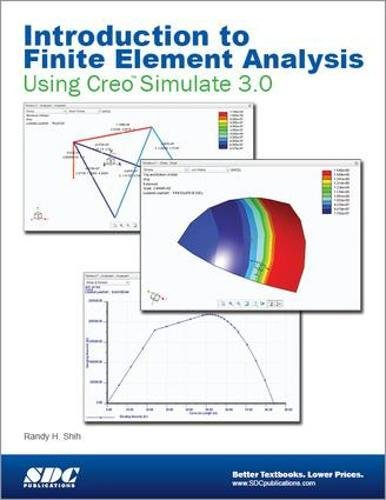# Introduction to Finite Element Analysis Using Creo Simulate 3.0Type
Book
Authors
ISBN 10
1585039152
ISBN 13
9781585039159
Category
Unknown  [ Browse Items ]
Edition

Publication Year
2014
Publisher
Pages
431
Abstract

Description
The primary goal of Introduction to Finite Element Analysis Using Creo Simulate 3.0 is to introduce the aspects of finite element analysis (FEA) that are important to the engineers and designers. Theoretical aspects of finite element analysis are also introduced as they are needed to help better understand the operations. The primary emphasis of the text is placed on the practical concepts and procedures of using Creo Simulate in performing Linear Statics Stress Analysis; but the basic modal analysis procedure is covered. This text is intended to be used as a training guide for both students and professionals. This text covers Creo Simulate 3.0 and the lessons proceed in a pedagogical fashion to guide you from constructing basic truss elements to generating three-dimensional solid elements from solid models. This text takes a hands-on exercise intensive approach to all the important Finite Element Analysis techniques and concepts. This textbook contains a series of twelve tutorial style lessons designed to introduce beginning FEA users to Creo Simulate. The basic premise of this book is the more designs you create using Creo Simulate, the Better you learn the software. With this in mind, each lesson introduces a new set of commands and concepts, building on previous lessons. Table of Contents Introduction 1. The Direct Stiffness Method 2. Truss Elements in Two-Dimensional Spaces 3. 2D Trusses in MS Excel and the Truss Solver 4. Creo Simulate Two-Dimensional Truss Analysis 5. Three-Dimensional Truss Analysis 6. Basic Beam Analysis 7. Beam Analysis Tools 8. Statically Indeterminate Structures 9. Two Dimensional Solid Elements 10. Three Dimensional Solid Elements 11. Axisymmetric and Thin Shell Elements 12. Dynamic Modal Analysis Index - from Amzon
Biblio Notes

Number of Copies